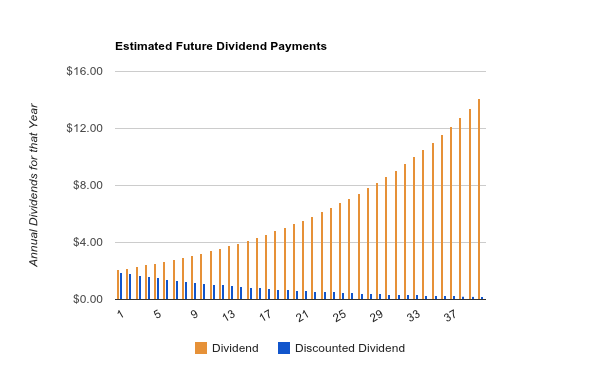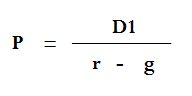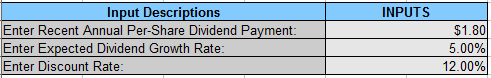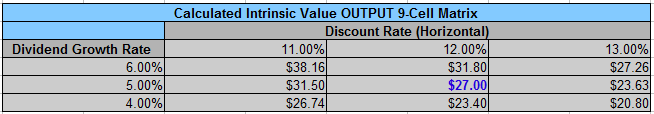Saturday, October 18, 2014

Dividend Discount Model Overview

The Dividend Discount Model (DDM) is the key valuation technique for dividend stocks.
The most straightforward form of it is called the Gordon Growth Model. This guide explains how it works and the streamlined way to use it.
Determining the fair value of a company means using Discounted Cash Flow Analysis (DCFA). DCFA, put simply, states that the present value of a company is equal to the sum value of all future cash flows that the company produces. But each future cash flow must be discounted to translate it into today’s dollars. This is logical: the purpose of a business is to produce cash flows, so the value of the business is equal to the sum value of all future discounted cash flows.
By discounted, what I mean is that due to the time value of money, a payment in the future is worth less than the same payment today. For example, if you can earn a 10% rate of return on your money over time, then a payment of \$10,000 one year from now would only be worth \$9,091 to you today, because if you had \$9,091 today, you could invest it at a 10% rate of return and turn it into \$10,000 a year from now. (\$9,091 multiplied by 1.10 equals \$10,000) So, the discounted version of, or the net present value of, \$10,000 one year from now, is equal to \$9,091.
Similarly, if you were to receive \$10,000 in five years, then this sum would only be worth \$6,209 to you today, because you could take \$6,209 and compound it by 10% annually to get \$10,000. (\$6,209 multiplied by 1.10 five times in a row equals \$10,000). So, the discounted version of, or the net present value of, \$10,000 five years from now, is equal to \$6,209.
To value a business, you would take the discounted values of all future annual expected cash flows, sum them together, and that’s the fair value of the business. You’re trading a present sum of money (the fair value), for a future series of expected cash flows, but each cash flow has to be translated into today’s value to take into account the time value of money and your target rate of return on your current money.
The inputs you need are the current free cash flow figures, the projected growth rate of those cash flows, and your target rate of return to use as the discount rate.
Obviously there’s a mix of art and science involved here. If appropriate inputs (expected cash flows) are used, the output (current intrinsic value) is objective. But since the inputs are future expected cash flows, there is uncertainty in those figures and it requires reasonably accurate estimates to be useful.

Enter the Dividend Discount Model

You can take that same approach, and tailor it specifically for analyzing a stock that pays good dividends, and this is the Dividend Discount Model. It’s also called the Dividend Growth Model, and the most straightforward form is called the Gordon Growth Model.
The DDM is based on the exact same idea, except that the share of stock represents what we’re valuing, and all future dividends represent all future cash flows of that share. The value of the stock is equal to the sum of the net present value of all future dividends.
For example, let’s say you’re analyzing a share of stock that pays \$0.50 in dividends per quarter, or \$2.00 per year. Furthermore, it’s a dividend aristocrat that has raised the dividend consecutively every single year for 25 years or more. You look over its history, and find that it has increased the dividend by an average of 8% per year over the course of several decades, but that the growth is slowing down. So, you estimate that the dividend will continue to grow by an average of only 5% per year going forward.
If you desire an 11% rate of return on your money, which would represent pretty good returns, then you can use that as your discount rate. So for example, when the \$2 in dividends goes up to \$2.10 next year (because it grew by 5%), this \$2.10 is only worth \$1.89 to you today, because if you had \$1.89 today, then you could turn it into \$2.10 in a year if you could compound it by 11% during that period.
The following chart shows the estimated value of dividend payments over the next forty years.The orange values are the actual dividends you expect to be paid if the dividend grows by 5% per year. The blue values are the discounted versions of those dividends; the dividends translated into today’s value based on your discount rate of 11%. As can be seen, if this chart continues forever, the sum of all dividends would be infinite, but the sum of all discounted dividends is finite, because the discount rate is larger than the dividend growth rate.
To calculate the fair value of this stock, we need to sum up all of those discounted dividends. It can be done with fancy math, but after a number of mathematical cancellations, the accurate equation is extremely simple, and this is called the Gordon Growth Model:In the formula, P is the fair price of the stock. D1 is next year’s expected dividend, which would be \$2.10 in this case. r is the discount rate, which is 1.11, and g is the dividend growth rate, which is 1.05.
When you plug everything into the equation, you get \$35. That’s the fair value of this hypothetical stock, assuming that the current dividend of \$2.00 really grows at that 5% per year into the future, and assuming an 11% rate of return is desirable to you. The math shows that it would be fair to trade \$35 in present value for the sum value of all future dividends, because when they are discounted by 11% per year, the sum of all of them is \$35.
If your estimates are correct, you’ll get a nice double-digit long-term rate of return.

Shortcomings

The first flaw of the Gordon Growth Model is that it assumes a constant dividend growth rate; it’s a constant growth model. This may be an acceptable estimate for a fairly high-yielding mature company, but for stocks with lower dividend yields and higher dividend growth, this may not be appropriate.
To fix this, you can move towards a more general two-stage or multi-stage Dividend Discount Model. The math gets a bit more tedious at this point, but a multi-stage DDM allows you to estimate that the dividend will grow at a certain rate for a number of years, and then slow down to another growth rate after that.
The second flaw of both the Gordon Growth Model and the whole Dividend Discount Model is that it’s quite sensitive to the accuracy of the inputs. Because dividend growth rates tend to be fairly high (higher than core company net income growth usually, due to share buybacks), even minor variances between the estimated dividend growth and the real dividend growth. This is why you should always have a margin of safety in your estimates. This also means that the DDM tends to be better for high yielding dividend stocks with lower dividend growth, rather than lower yielding stocks with higher dividend growth rates.

The one product I offer on this site is the Dividend Toolkit, which is a comprehensive stock guide that also comes with an easy-to-use valuation spreadsheet to calculate the fair price for dividend stocks.
Specifically, it’s a spreadsheet tool that lets you put in a single set of inputs, and then it automatically calculates a range of fair values based on different outcomes.
See, all valuation methods rely on estimated inputs. The two main things you need are an estimate for the growth rate, and your discount rate. (Your discount rate should be your target rate of return.) But if those inputs are off even slightly, the whole valuation method will be off. With basic freebie valuation calculators, you need to put in more than one set of inputs if you want to see several output options, and you have no way of keeping track of the differences.
Suppose you want to calculate the fair value of a stock using the Dividend Discount Model (which is explained in significantly more detail in the book), and you estimate that the dividend will grow by 5% per year, and you’re using 12% as your discount rate. First, you put the simple inputs into the Dividend Discount Model spreadsheet tool:And the tool instantly updates the output chart to tell you the fair value of the stock:This output chart will not only tell you the fair stock value based on those inputs, but will also tell you the fair stock value based on nearby inputs. In this example, in addition to calculating the results for 5% dividend growth and a 12% discount rate, it will automatically show what the fair value is if it turns out that the stock only grows its dividend by 4%, or if you use a discount rate of 11% instead.
This particular tool provides nine total output values, centered around the primary estimate. In this example, because I chose 5% for my estimated dividend growth, the output chart automatically adjusts to show the calculated fair values for 4%, 5%, and 6% growth. And because I chose 12% for my discount rate, the output chart automatically adjusts to show 11%, 12%, and 13% discount rates.
So there are nine total outputs. In this example, I was looking to estimate the value of this stock that paid \$1.80 in dividends with estimates for 5% annual dividend growth and a 12% discount rate. The primary result is the \$27.00 figure in the middle, which corresponds to 5% growth and 12% target rate of return. The result in the top left corner shows a related estimate, except that it shows the fair value for the same stock but with a 6% dividend growth rate and with a less aggressive 11% discount rate. The result in the bottom right corner shows another estimate, except that it shows the fair value if the stock only has a 4% dividend growth rate and if a 13% discount rate is used. The other cells show other combinations in between those two extremes.
Take a look at the Dividend Toolkit if you want that tool, or read the reviews. Hundreds of investors use it now. The guide covers how to make reasonable estimates for dividend growth as well.
Either way, make sure you’re signed up for the monthly dividend newsletter, at no cost.

Recent Posts From DIV-Net Members

• - I have been sitting on the sidelines of option trading for a while, researching the subject and educating myself and seeing how best to use this as a mediu...
14 hours ago
• - For Anyone Thinking About Moving To Maryville, Tennessee Eastern Tennessee has become a popular destination. So, let’s take a close look at the pros and ...
16 hours ago
• - It is difficult to watch your portfolio value slump when the market is selling off. I am down almost \$7k as of today. But I am not worried about it. Why?...
1 day ago
• - My monthly *7 Dividend Growth Stocks* series present seven high-quality dividend growth stocks for further analysis and possible investment. I apply diffe...
1 day ago
• - If your goal is to accumulate wealth for a comfortable retirement, then there is no risk-free path. Throughout time every angle has been tried and failed. ...
2 days ago
• - Last year was a record for dividend payments in the US. This continues the trend of the past 13 years, which wasn't interrupted even in 2020. I obtained da...
2 days ago
• - Wow, I can’t believe it. I once again find myself in credit card debt. I never thought that I would have to tackle this beast, but here we are. For the m...
2 days ago
• - Great report JC. Congrats on cross \$1300 for the first time. Also, I really like how you break down your report into dollars earned per hour. That's really...
1 week ago
• - Welcome to our December 2021 Passive Income report. This is where I track our investment income from dividend stocks, index funds and exchange-traded fu...
1 week ago
• - The markets have been in turmoil and I have seen my investments drop by 20%. My standard reply to market volatility has been that I don’t care because I ...
7 months ago
• - I am a little shocked, and disappointed in myself, that it has been almost eight months since my last post. The timing makes sense though: I went back to s...
8 months ago
• - Once a month, I like to talk about my total passive income for the previous month. I do this to track how much passive income is coming in. When I start ...
1 year ago
• - It is time to give a new update about my current portfolio. April has shown some recovery of my portfolio and also of my dividend … The post Portfolio Up...
1 year ago
• - Note: This page contains affiliate links for certain services and products. I may receive compensation at no additional cost to you when you click on thes...
2 years ago
• - Today I added a new position: ABBV. They didn't show up on my screener because some spin-off activity made it look like their div history is much shorter t...
4 years ago